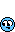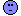# Discussion for May 10 draw

#### dwoods99

##### Member
Opening a thread here to split discussion vs picks for the \$24 million draw on Friday#### Dennis Bassboss

##### Member
Great idea Dwoods...that way we won't bury the players picks...#### Dennis Bassboss

##### Member
Lets start by looking at the Double announcers...
-------------With bonus––---------------
The ways the numbers showed up....
the last-----------last------------last-------
170 draws------25 draws-----10 draws--

22=39 times---46=08 times--30=05 times
37=38 times---34=08 times--35=04 times
10=37 times---04=08 times--34=04 times
46=36 times---37=07 times--26=04 times
08=36 times---22=07 times--16=04 times
04=36 times---16=07 times--37=03 times
33=35 times---10=07 times--27=03 times
05=35 times---02=07 times--22=03 times
34=33 times---29=06 times--04=03 times
30=33 times---28=06 times--02=03 times
06=33 times---26=06 times--46=02 times
39=32 times---11=06 times--29=02 times
25=32 times---05=06 times--28=02 times
16=32 times---43=05 times--25=02 times
21=31 times---31=05 times--17=02 times
26=30 times---30=05 times--15=02 times
23=30 times---27=05 times--12=02 times
02=30 times---23=05 times--11=02 times
43=29 times---17=05 times--10=02 times
36=29 times---08=05 times--08=02 times
31=29 times---06=05 times--06=02 times
27=29 times---41=04 times--05=02 times
42=28 times---39=04 times--01=02 times
32=28 times---36=04 times--47=01 time
29=28 times---35=04 times--45=01 time
28=28 times---21=04 times--44=01 time
19=28 times---20=04 times--43=01 time
03=28 times---14=04 times--42=01 time
01=28 times---13=04 times--40=01 time
40=27 times---45=03 times--39=01 time
35=27 times---38=03 times--38=01 time
24=27 times---32=03 times--36=01 time
17=27 times---25=03 times--32=01 time
12=27 times---18=03 times--31=01 time
38=26 times---15=03 times--24=01 time
45=25 times---12=03 times--23=01 time
11=25 times---09=03 times--20=01 time
07=25 times---03=03 times--18=01 time
44=24 times---44=02 times--13=01 time
20=24 times---40=02 times--09=01 time
09=24 times---01=02 times--03=01 time
15=23 times---47=01 time---41=00 time
13=23 times---42=01 time---33=00 time
41=22 times---33=01 time---21=00 time
18=22 times---24=01 time---19=00 time
47=21 times---19=01 time---14=00 time
14=21 times---07=01 time---07=00 time

-------------Without bonus–--------------
The ways the numbers showed up....
the last-----------last------------last-------
170 draws------25 draws-----10 draws--

05=34 times---22=07 times--35=04 times
37=33 times---16=07 times--16=04 times
22=33 times---10=07 times--37=03 times
10=33 times---02=07 times--34=03 times
25=31 times---46=06 times--30=03 times
46=30 times---37=06 times--27=03 times
33=30 times---34=06 times--26=03 times
21=30 times---28=06 times--22=03 times
16=30 times---05=06 times--02=03 times
08=30 times---43=05 times--29=02 times
06=30 times---31=05 times--28=02 times
39=29 times---29=05 times--25=02 times
34=29 times---27=05 times--17=02 times
30=28 times---26=05 times--15=02 times
04=28 times---11=05 times--12=02 times
31=27 times---08=05 times--10=02 times
32=26 times---39=04 times--08=02 times
29=26 times---36=04 times--06=02 times
27=26 times---35=04 times--05=02 times
24=26 times---23=04 times--01=02 times
23=26 times---21=04 times--47=01 time
02=26 times---20=04 times--46=01 time
01=26 times---17=04 times--45=01 time
43=25 times---14=04 times--44=01 time
38=25 times---06=04 times--43=01 time
36=25 times---45=03 times--40=01 time
19=25 times---41=03 times--39=01 time
03=25 times---38=03 times--38=01 time
35=24 times---32=03 times--36=01 time
26=24 times---30=03 times--32=01 time
28=23 times---25=03 times--31=01 time
17=23 times---15=03 times--24=01 time
40=22 times---12=03 times--23=01 time
20=22 times---09=03 times--20=01 time
11=22 times---04=03 times--13=01 time
45=21 times---03=03 times--11=01 time
42=21 times---40=02 times--09=01 time
12=21 times---18=02 times--04=01 time
09=21 times---13=02 times--03=01 time
07=21 times---01=02 times--42=00 time
44=20 times---47=01 time---41=00 time
15=20 times---44=01 time---33=00 time
13=20 times---33=01 time---21=00 time
47=19 times---24=01 time---19=00 time
18=19 times---19=01 time---18=00 time
14=19 times---42=01 time---14=00 time
41=16 times---07=01 time---07=00 time

What is different than my usual grid is that these are the announcers formed by all the possibles pair that you can make out of the last winning set....They are announcing millions here..lol..lol..lol
\$\$\$\$\$\$\$
To add to the drama..here are the numbers from the last six draws bonus included....
01-02-05-06-08-09-10-12-13-15-16-17-18-19-20-22-
24-26-27-28-29-30-31-34-35-37-38-42-43-45-47
Many should be in there....

O:K: After knowing the results of tonight's draw and if we would have mix the two theory look at that....
We know that the numbers announced 1 or 0 times comes up the most...so if we take the numbers from the last 6 draws and get rid of all the numbers that are announced more than 1 and 0 time from that list we wind up with this...
09-13-18-19-20-24-31-38-42-43-45-47 ...interesting....

Last edited:

#### Picard

##### Member
Ok, here's the big question.

Will 38 show up again???What's with that number. It's wrecking my wheels.
I hope it dosn't show!

I'd say the sum should be high, around 150-180.

#### Dennis Bassboss

##### Member
Picard said:
Ok, here's the big question.

Will 38 show up again???What's with that number. It's wrecking my wheels.
I hope it dosn't show!

I'd say the sum should be high, around 150-180.

I'll bet high too!Dam 38...go figure...starting to reminds me of the 02.....According to the announcers grid ...38 is still in the striking zone.....could hit again....Last edited:

#### Picard

##### Member
Interesting.....

I see from LottoPro, it hasn't hit more than twice in a row.
Well, except the recent draws.

Last edited:

#### Dennis Bassboss

##### Member
I have these hits for 38 in Lotto-Master..it has hit in draws (bonus included)...
1-6-7-9-10-11-12-23-38-39-44-50-51-54-63-64-67-69-70-84-89-103-120-126-134-135-139-140-148-153-155-156-162-175-181-197-202-206-212-217-219-220-234-237-239-252-273-289-301-307-312-321-323-326-328-348-356-369-374-389-411-412-413

#### Dennis Bassboss

##### Member
41 is so overdue here ....that number is another strange one and according to the announcers grid it should strike....but it has been like that at times recently and it still......but I'll put it in my set...LDs 01 might pay us a visit!

#### Beaker

##### Member
The number I see a lot of hits for is 40

Something will happen around 38-39-40-41

Consider that 38There is good history for 4peats.I like LD 0 and 2

#### Dennis Bassboss

##### Member
The 30-40 looks good...I like the 14 also...39-40 or 29-30 make a triple and you have 41 or 31...or both...16 is also in striking distance....with 46???

Last edited:

#### Beaker

##### Member
The 5 looks good also - 5 with 45 to repeat#### Dennis Bassboss

##### Member
From an announcing point of view here are the last 25 announced draws from pairs....
You'll see who was hot in the latest announced draws....
draw/
356--03 10 17 33 36 38 46 (04)
357--02 04 11 14 16 23 28 (17)
359--02 10 14 16 21 22 27 (46)
360--03 05 11 26 28 43 46 (06)
364--18 22 28 31 40 41 45 (37)
367--21 27 34 36 37 39 41 (13)
368--02 05 06 10 11 14 29 (23)
370--12 14 19 29 37 41 43 (34)
375--11 21 31 32 34 39 43 (41)
378--06 20 22 23 28 37 46 (07)
384--05 09 10 18 20 23 46 (04)
386--08 15 16 17 22 32 39 (04)
389--08 13 21 36 38 45 46 (29)
390--04 08 10 20 25 26 31 (44)
393--02 05 09 29 31 34 43 (13)
394--08 16 22 32 37 44 46 (11)
396--25 26 30 34 37 40 47 (04)
398--06 09 13 25 27 30 45 (26)
399--01 02 15 27 30 31 34 (04)
402--06 11 23 27 35 39 43 (30)
403--05 15 16 17 28 29 35 (34)
407--02 03 04 12 26 35 36 (46)
409--02 10 22 24 26 34 37 (18)
410--01 10 16 17 22 29 35 (30)
412--05 08 12 16 20 28 38 (42)

the last one is the latest...

Last edited:

#### Dennis Bassboss

##### Member
Beaker said:
The 5 looks good also - 5 with 45 to repeatGood point Beaker! The 05 does look very good! But it has hit in the last announced drawhowever the 30 is a good substitute here..if you look closely last time 45 hits in this announced draws...30 was also there...The 10 has hit three time in a row lately(regular database) and twice after beeing the bonus like in the last draw...it is that kind of number too!Last edited:

#### Beaker

##### Member
Dennis, one thing I'm doing to explore this "history in history" concept - maybe you've already done this - but I'm taking the announced history and running the last draw from that history again. I guess what I'm getting is the counts of the best "Announcers of the Announcers" - the AA numbersSo, I took 5-8-12-16-20-28-38-42 and run them on the 49 3 number announced draws. Maybe if you keep going through these iterations you could narrow in on something.I guess you could also do this for the 170-2 number announnced draws.

Draws 148 and 315 are interesting#### Beaker

##### Member
Dennis Bassboss said:

Good point Beaker! The 05 does look very good! But it has hit in the last announced drawDon't forget they act like normal draws - you need some from that draw as repeaters#### Dennis Bassboss

##### Member
Interesting Beaker ...I will try ....but what I had in mind was to compare the double-triple grid send this package to goswinus and catch the best performing set....after a while I'm sure that we would catch 4 or 5 more regularly by adding some numbers to it...#### Beaker

##### Member
Dennis Bassboss said:
Interesting Beaker ...I will try ....but what I had in mind was to compare the double-triple grid send this package to goswinus and catch the best performing set....after a while I'm sure that we would catch 4 or 5 more regularly by adding some numbers to it...That approach is goodThe sample is so small with 3 that the 2 number is good.

Funny how 2 of the numbers in the last draw are in almost half the draws#### Dennis Bassboss

##### Member
Beaker said:

Don't forget they act like normal draws - you need some from that draw as repeatersI think that the 16 and the 42 will take care of that...#### Beaker

##### Member
Dennis Bassboss said:

I think that the 16 and the 42 will take care of that...I was thinking 5-12#### Dennis Bassboss

##### Member
Don't forget that elusive 07 Beaker....It is in the radar screen...